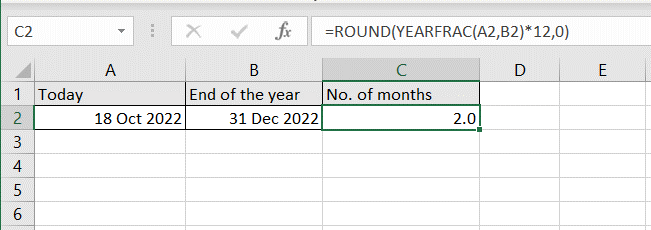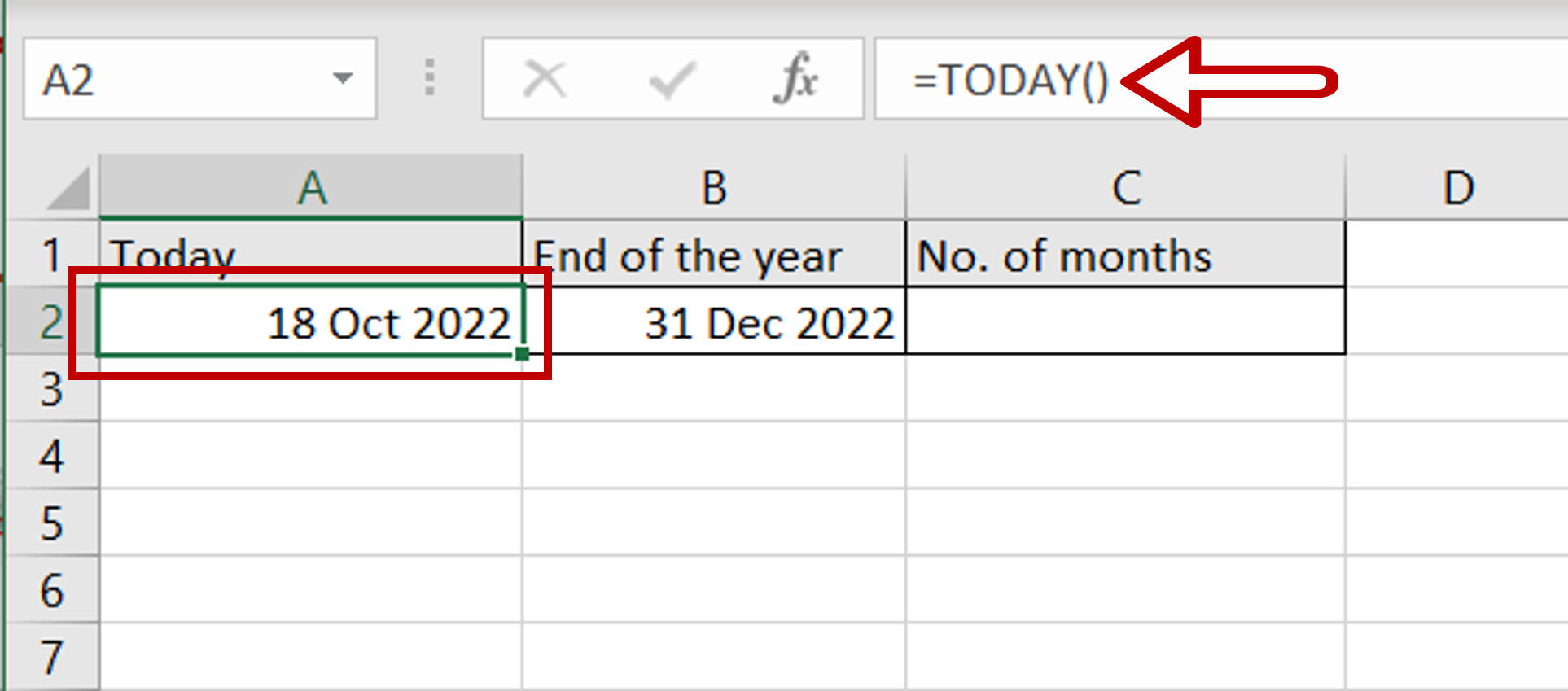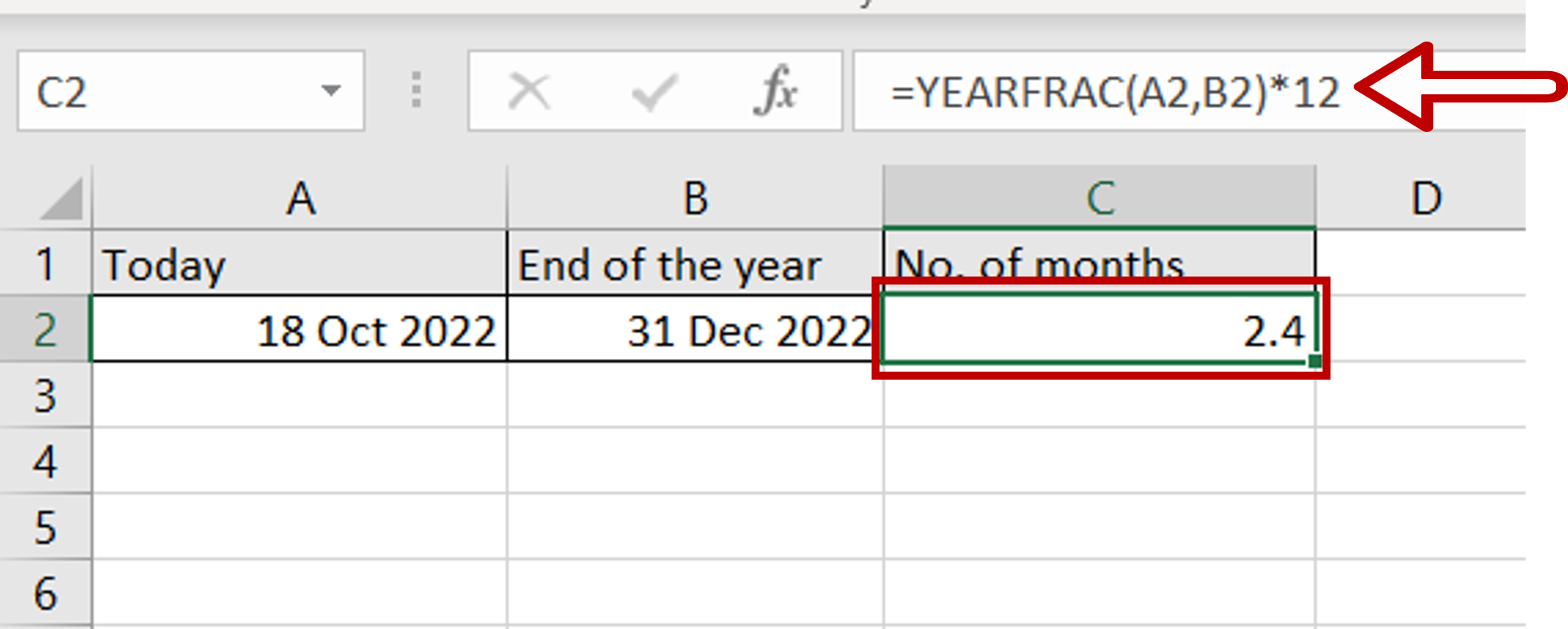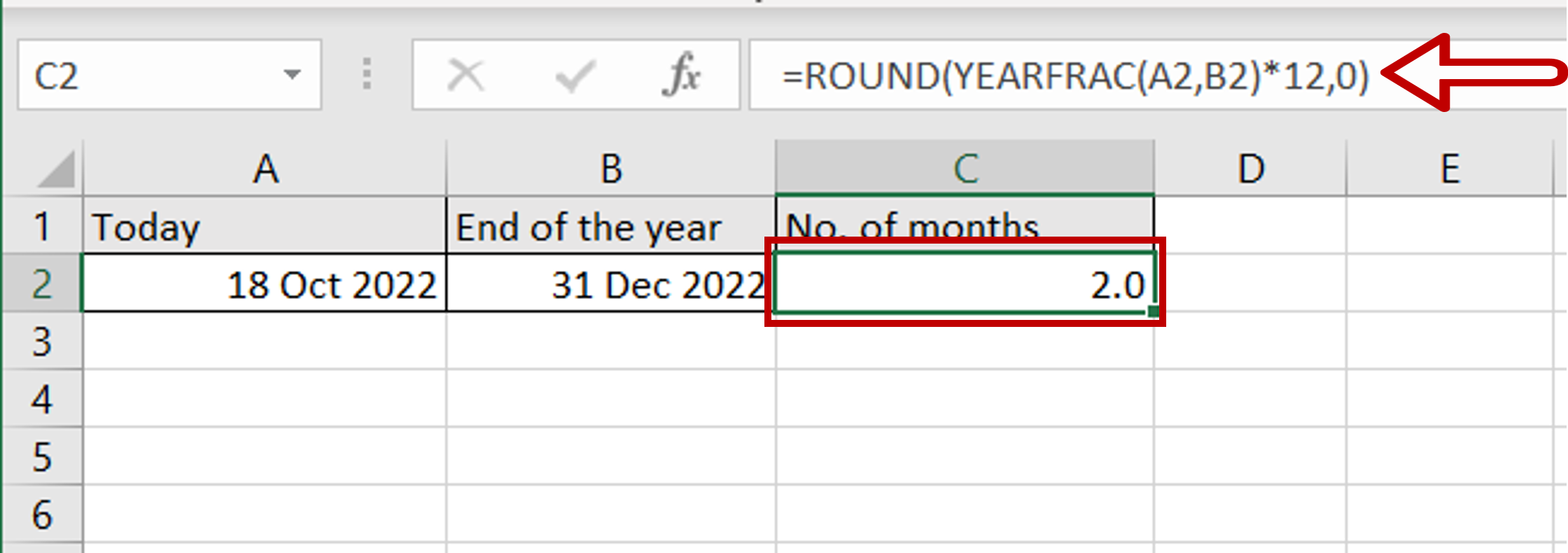# How many months are left in the year

You can watch a video tutorial here.

It is simple to find how many months are left in the year using Excel. When monitoring the progress of a project, you may want to compute the number of months left in the year so that you can estimate the workload required to achieve a certain target. There is no direct function in Excel to compute this, but there is a workaround using the YEARFRAC() function.The YEARFRAC() function returns a number that represents the fraction of the year between 2 dates. This number can be multiplied by 12 to get the number of months. The syntax is:

YEARFRAC(start_date, end_date, [basis])

>start_date: the date from which the count is to begin
>end_date: the date at which the count ends
>basis (optional): the type of day count to use

Note: The start and end dates have to be formatted as dates, not text, else the function will not work properly.

### Step 1 – Set up the dates=TODAY()
– Enter the date for the end of the year

### Step 2 – Create the formula– Type the formula using cell references:
= YEARFRAC(Today, End of the year)*12
– Press Enter
– A fraction representing the number of months left in the year is displayed

### Step 3 – Enhance the formula to round off the number– If you need to use a whole number and not a decimal, enhance the formula using the ROUND() function:
= ROUND(YEARFRAC(Today, End of the year)*12,0),0)
– The number will be rounded up or down to the nearest whole number